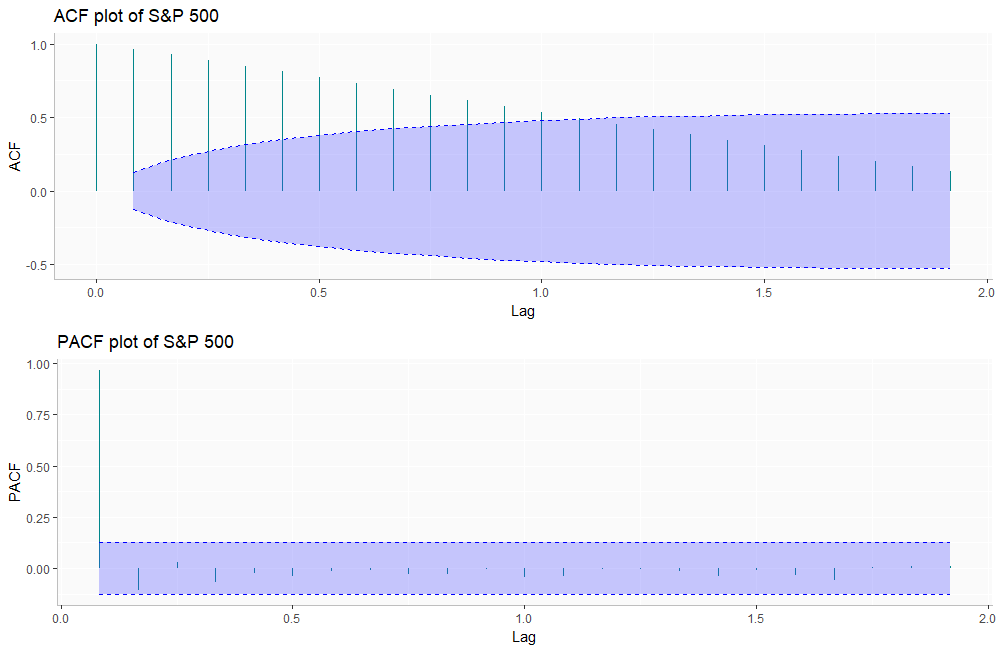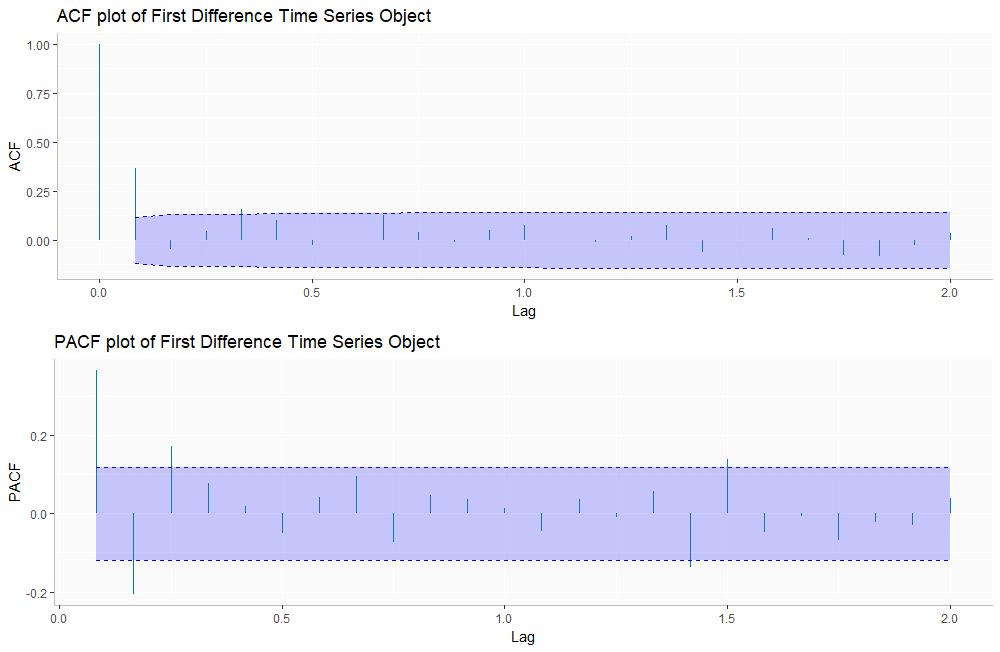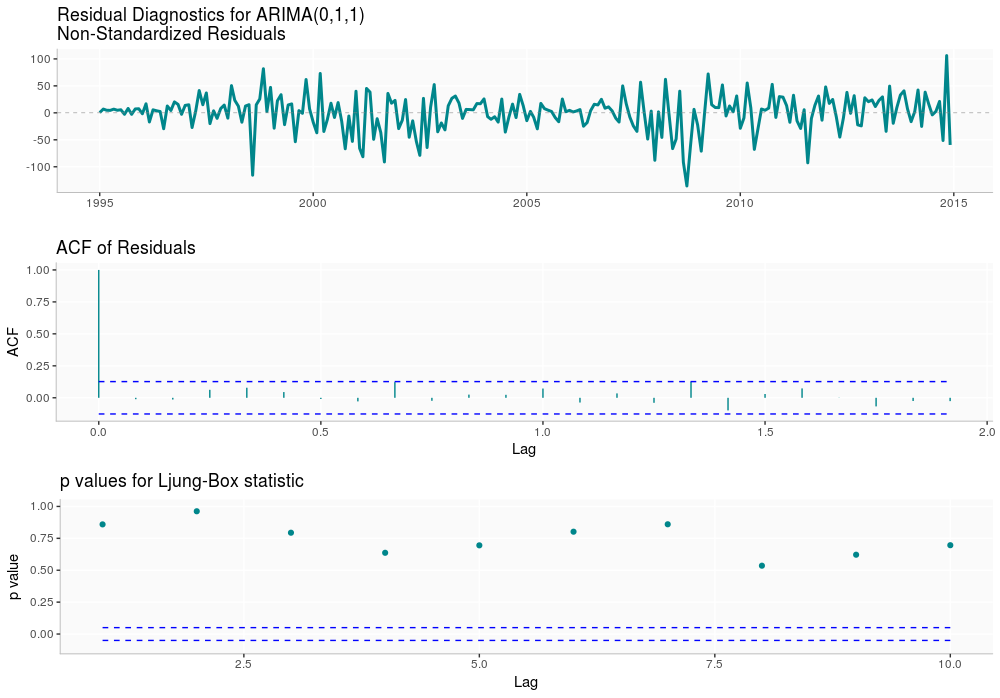# Performing a Time-Series Analysis on the S&P 500 Stock IndexTime-series analysis is a basic concept within the field of statistical learning that allows the user to find meaningful information in data collected over time. To demonstrate the power of this technique, we'll be applying it to the S&P 500 Stock Index in order to find the best model to predict future stock values.

Throughout this tutorial, we'll leverage the horse-power of RStudio and deliver, where appropriate, gorgeous interactive data visualizations using ggplot2 and plotly. Just to be clear, using a time-series analysis to invest in stocks is highly discouraged. We've chosen to predict stock values for the sake of example only.

The GitHub repository you'll need to follow this tutorial is located here

First, to correctly load the packages we'll be using in this tutorial into your RStudio console, select File, Open Project in New Session, and click on the preexisting R Project in the repo. With the `packrat` package, the `.Rprofile` script will automatically run files in the `packrat` directory and load the appropriate packages into the R environment.

Once this is complete, you should have a new RStudio session. Here we will be referencing the `helper_functions` script, which contains all the necessary packages. We use:

``````source(here("src",'helper_functions.R'))
``````

For this demonstration we will load them in manually:

``````# LOAD YOUR PACKAGES
library(ggplot2)
library(forecast)
library(plotly)
library(ggfortify)
library(tseries)
library(gridExtra)
library(docstring)
library(here)
here() # Should output current work directory
``````

Using the `here` package, we are going to set the working directory. We'll be able to reference different subdirectories to make the workflow easier.

Get Data

Now we collect our data. We want to use reliable sources of complete and accurate data. For the purposes of this tutorial, we collected 21 years (1995-2015) of S&P 500 Stock Index data at a monthly frequency (a total of 252 observations) from Yahoo Finance, which you can download here. We chose to use the Adjusted Closing Value for this analysis.

We must include our data set within our working R environment. For this we use:

``````data_master <- read.csv(here("data", "data_master_1.csv"))

attach(data_master)
``````

Now we can call our S&P 500 Stock Index data by typing `data_master\$sp_500` into our terminal.

Helper Functions

For this project, we created a helper script which contains functions that automate many of our plots.

In order to provide well-written documentation for these functions, we utilized the `docstrings` package which allows you to view them on RStudio:

``?function_name``

Exploratory Analysis

Now, we want to get a feel for our data in order to decide which models may be appropriate for our forecast. To do this, we will plot our data and diagnose for trend, seasonality, heteroskedasticity, and stationarity. We will go over these concepts in further detail below.

Create a Time-Series Data Object

Our S&P 500 Stock Index data is in the form of a time series; this means that our data exists over a continuous time interval with equal spacing between every two consecutive measurements.

In R we are able to create time-series objects for our data vectors using the `ts()` method. Select the vector you would like to use as the first argument, and tune the `start` and `freq` (frequency) parameters. Then output the time-series data to the terminal by calling your newly-created time-series object.

``````sp_500 <- ts(data_master\$sp_500, start=c(1995, 1), freq=12)
``````

Here, we use our own function called `plot_time_series`, which does as its name suggests:

``plot_time_series(sp_500, 'S&P 500')``

Before you begin any analysis, you will be splitting the data to remove 2015 to use as a test set.

``sp500_training <- ts(sp_500, start=c(1995, 1), end=c(2014, 12), freq=12)``

Plot a Time Series

Plotting data is arguably the most critical step in the exploratory analysis phase. (We chose to emphasize the time-series object that has intervals from 1995 to 2014, a choice we will explain later!) Visualizing our time-series data enables us to make inferences about important components, such as trend, seasonality, heteroskedasticity, and stationarity. Here is a quick summary of each:

• Trend: We say that a dataset has a trend when it has either a long-term increase or decrease.

• Seasonality: We say that a dataset has seasonality when it has patterns that repeat over known, fixed periods of time (e.g. monthly, quarterly, yearly).

• Heteroskedasticity: We say that a data is heteroskedastic when its variability is not constant (i.e., its variance increases or decreases as a function of the explanatory variable).

• Stationarity: A stochastic process is called stationary if the mean and variance are constant (i.e., their joint distribution does not change over time).

We start our analysis by plotting our time series object to give us a visual basis to start our modeling.

`````` plot_time_series(sp500_training, 'S&P 500')
``````

You can easily see that our time series has instances of both positive and negative trend. Overall, it is very volatile, which tells us that we will have transform the data in order for the Box-Jenkins Methodology to predict with better accuracy.

Test for Stationarity

We will utilize a few statistical methods to test for stationarity. We must be wary of our model having a unit root; this will lead to non-stationary processes.

``````Box.test(sp_500, lag = 20, type = 'Ljung-Box')
``````
Our output:
``````> Box.test(sp500_training, lag = 20, type = 'Ljung-Box')

Box-Ljung test

data:  sp500_training
X-squared = 2024.8, df = 20, p-value < 2.2e-16
``````

Now we will utilize the Augmented Dickey-Fuller Test for stationarity. The null hypothesis states that large p values indicate non-stationarity and smaller p values indicate stationarity. (We will be using 0.05 as our alpha value.)

``adf.test(sp_500)``

### Here's our output:

``````> adf.test(sp500_training)

Augmented Dickey-Fuller Test

data:  sp500_training
Dickey-Fuller = -1.7877, Lag order = 6, p-value = 0.6652
alternative hypothesis: stationary
``````

You can see our p value for the ADF test is relatively high. For that reason, we need to do some further visual inspection — but we know we will most likely have to difference our time series for stationarity.

Decompose a Time Series

Beyond understanding the trend of your time series, you want to further understand the anatomy of your data. For this reason, we will break down our time series into its seasonal component, trend, and residuals.

``````plot_decomp(sp500_training, 'S&P 500')
``````

The trend line shows us what we already know; we can see there might be some seasonality in our time-series object.

Model Estimation

### Diagnosing the ACF and PACF Plots of Our Time-Series Object

ACF stands for "autocorrelation function" and PACF stands for "partial autocorrelation function." The ACF and PACF diagnosis is employed over a time-series to determine the order in which we are going to create our model using ARIMA modeling. Loosely speaking, a time series is stationary when its mean, variance, and autocorrelation remain constant over time.

These functions help us understand the correlation component of different data points at different time lags. Lag refers to the time difference between one observation and a previous observation in a dataset. Let's examine our plots!

``````# DIAGNOSING ACF AND PACF PLOTS
plot_acf_pacf(sp500_training, 'S&P 500')
``````When there is large autocorrelation within our lagged values, we see geometric decay in our plots. This is a huge indicator that we will have to take the difference of our time series object.

### Transform Data to Adjust for Non-Stationarity

Based on our visual inspection of the time-series object and the statistical tests used for exploratory analysis, it is appropriate to difference our time-series object to account for the non-stationarity. Let's see how the object fares!

A way to make a time series stationary is to find the difference across its consecutive values. This helps stabilize the mean, thereby making the time-series object stationary.

For this we use the `diff()` method.

``````tsDiff <- diff(sp500_training)
``````

Next we plot our transformed time series:

``````plot_time_series(tsDiff, 'First Difference')
``````

This plot suggests that our working data is stationary. You will want to confirm this by running the same tests and looking at the ACF and PACF diagnostics over the differenced data to find out if you can proceed to estimating a model.

### Test for Stationarity

Let's apply the same tests to our differenced time-series object:

``````> Box.test(tsDiff, lag = 20, type = 'Ljung-Box')

Box-Ljung test

data:  tsDiff
X-squared = 58.2, df = 20, p-value = 1.347e-05
``````

Now let's use the ADF test:

``````> adf.test(tsDiff)

Augmented Dickey-Fuller Test

data:  tsDiff
Dickey-Fuller = -4.9552, Lag order = 6, p-value = 0.01
alternative hypothesis: stationary

Warning message:
In adf.test(tsDiff) : p-value smaller than printed p-value
``````

This cryptic warning message means that the result yields a small p value, which makes us reject the null suggestion stationarity.

Diagnose the ACF and PACF of a Transformed Time-Series Object

The plot below helps us confirm that we have stationarity and helps us decide which model we will use. It is important to keep in mind that we have a difference parameter equal to one (i.e., d = 1) because of the previous transformation we carried out.

``````plot_acf_pacf(tsDiff, 'First Difference Time Series Object')
``````From the above plots, we deduce that an MA(1) model (where MA stands for moving average) best fits our data because the ACF cuts off at one significant lag and the PACF shows geometric decay.

Because we are examining the differenced time series, we have to use the combined model ARIMA (Autoregressive integrated moving average); thus, our model so far is ARIMA(0, 1, 1).

Build a Model

Our findings in the exploratory analysis phase suggest that model ARIMA(0, 1, 1) might be the best fit. Fortunately, there is a function in R that we can use to test our findings.

The `auto.arima()` method, found within the `forecast` package, yields the best model for a time series based on Akaike-Information-Criterion (AIC). The AIC is a measurement of quality used across various models to find the best fit. After running our original and differenced data sets through the `auto.arima()` method, we confirmed that the ARIMA(0, 1, 1) is our best fit model.

We use the `Arima()` method to fit our model and include our training data set, `sp500_training`as the first argument.

``````fit <- Arima(sp500_training, order = c(0,1,1),
include.drift = TRUE)
summary(fit)
``````

Here's the summary of our model (using the `summary()` method):

``````> summary(fit)
Series: sp500_training
ARIMA(0,1,1) with drift

Coefficients:
ma1   drift
0.5666  6.4975
s.e.  0.0551  3.4326

sigma^2 estimated as 1161:  log likelihood=-1181.58
AIC=2369.17   AICc=2369.27   BIC=2379.59

Training set error measures:
ME     RMSE      MAE
Training set -0.00911296 33.85289 24.84955
MPE     MAPE      MASE
Training set -0.00840343 2.141218 0.1310854
ACF1
Training set -0.01137429
``````

Our next step is to run residual diagnostics to ensure our residuals are white noise under our initial assumptions. For this we will use the `ggtsdiplay()` method.

``````# RESIDUAL DIAGNOSTICS
ggtsdiag_custom(fit) +
theme(panel.background = element_rect(fill = "gray98"),
panel.grid.minor = element_blank(),
axis.line.y = element_line(colour="gray"),
axis.line.x = element_line(colour="gray"))

residFit <- ggplot(data=fit, aes(residuals(fit))) +
geom_histogram(aes(y =..density..),
binwidth = 5,
col="turquoise4", fill="white") +
geom_density(col=1) +
theme(panel.background = element_rect(fill = "gray98"),
panel.grid.minor = element_blank(),
axis.line   = element_line(colour="gray"),
axis.line.x = element_line(colour="gray")) +
ggtitle("Plot of SP 500 ARIMA Model Residuals")
``````Based on our diagnostic plot, the residuals to seem to display a normal distribution.

This model appears to confirm all of our assumptions, which means we can continue to the forecasting phase!

Forecast

Since we believe we've found the appropriate model, let's begin forecasting! As you'll see, we relied quite heavily on the `autoplot()` function, since we couldn't find a way to add the actual values to the plot. The code for the workaround we used can be found in the project's Github Repository. You should run the `autoplot.forecast()` in order to get the plots we have here.

For now, we will create a time-series object that will include the actual 2015 values. In our function, it will be called up by the parameter `holdout`.

Next we use the `forecast` function, `ggplot2`and `plotly` to visualize the predictions for the year 2015! Within the plots, the forecasted values are BLUE, the actual 2015 values are in RED, the 80% confidence intervals are encompassed in the YELLOW bands, and 95% confidence intervals are encompassed in the ORANGE bands.

``````for_sp500_all <- forecast(fit, h = 12)
``````

Next, let's create the test set:

``sp500_test <- window(sp_500, 2015, c(2015, 12))``

Now let's plot the forecast and compare to the actual values for 2016:

We can see that the model performs well and within the 80% and 95% confidence intervals. You can forecast values even further into the future by tuning the appropriate parameters. Please not that this forecast project is for educational purposes only and we do not recommend investing based on predictions made during this project. The stock market is very volatile.

Conclusions

The forecasting method we used to find the best model receives the lowest MAE and MAPE. You can read more about this in "Evaluating Forecast Accuracy" by Rob J. Hyndman, which can be found here.

Below, we use the accuracy method that includes the test set to give us metrics for all models. The functions is called as follows:

``````# Using round function to make it more readable
round(accuracy(fit, sp500_test), 3)
``````

Here are the results for the test set (the function will return both training and test set metrics, but we're only concerned with the test set metrics):

Surprisingly, other models performed better on the test set than the ARIMA model. This could be a result of overfitting, since our test set was really small. After a few iterations, we have been able to expand the test set to include data up until 2017 on the repo and the original inertia7 project.

For the sake of the dashboard, we have decided to leave the data in their original state. We do, however, encourage that you explore the models with a larger test set.

Sources Cited

Below are some resources that we used to write this piece, and that we would highly recommend you read too! They all cover the material very well without getting overly technical. (Although, if you'd like something more in depth, we would recommend "Time Series Analysis and Its Application with R Examples," which can be found here). I would also like to acknowledge Rob J. Hyndman for his major contributions to time-series analysis and the R community with the creation of the `forecast` package, among other contributions, and Hadley Wickham for his contribution to both RStudio and `ggplot2 `— without these tools, none of this work would have been possible. Thank you.

Citations for Packages Used

Citations created using the function (in R):

``````citation(""packageName"")
``````

This post was a group effort. In addition to Raul Eulogio, whose information can be viewed below, the following people contributed their knowledge and creativity to this project:

David A. Campos

David is a startup and technology junkie with a passion for building products that help human beings reach their highest potential. Most recentlyDavid cofounded Inertia7, a social platform for computer and data science enthusiasts to share their open-source projects. Still in its early development, Inertia7 has attracted more than 30 contributors,100 open-source projects, and 9,000 users. David was also the founder of Napses, an EdTech startup that placed third in the 2013 New Venture Competition at University of California, Santa Barbara (UCSB). In his free time, he loves learning, coding, drumming, painting, hiking, music festivals, and hanging out with friends. He holds a B.A. in Economics and Spanish, as well as a Technology Management Certificate from UCSB.

Nathan Fritter

Nathan has a B.S. in Statistics from UCSB, and is currently working as a business inteligence analyst for PointCare in the SF Bay Area. He contributes to inertia7, a open source platform for data science projects, and was a very active member in the Data Science at UCSB club during his time as an undergrad. Nathan can be seen catching a Golden State Warriors game with a nice IPA, putting in a good workout at the local gym, or working hard on an interesting machine learning project.

Amil Khan

Amil is currently a full time student at UCSB studying statistics. He also works as a materials science researcher at UCSB Beyerlein Lab, using his skills in machine learning to further the mechanical performance of advanced materials. His specialties are data visualization, time series, scientific computing, and storytelling. In his free time, he works on his Jeep JK and watches Lakers basketball.

Kimberly Specht

Kim's background is in applied statistics at UCSB. Her interest in data science has led her to a career in healthcare analytics. She is now working at a not-for-profit healthcare system in the San Francisco Bay Area where she is hoping to help transform patient care with predictive analytics.